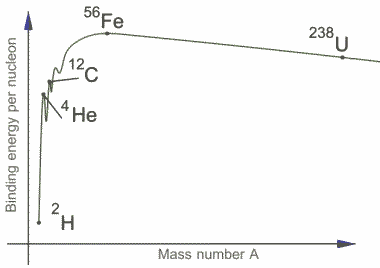# Q: Binding energy per nucleon & energy released in reaction

• I
• victorhugo
In summary, energy is released in nuclear reactions when the product atom requires more binding energy per nucleon than the original nucleus.f

#### victorhugo

EDIT: I figured out what I'm stuck on is WHY do nuclear reactions favor the a reaction such that the product has a HIGHER binding energy per nucleon? Also how is energy released in a fusion reaction if the product requires more binding energy per nucleon?

I need an explanation...

"The graph shows the binding energy per nucleon. Using this diagram, explain how energy may be released in a nuclear reaction." (graph on the original question is energy in MeV vs Atomic Number)Their sample answer is "The binding energy of a nucleon is a measure of the strength of the bond between a nuclear force and the nucleus. For a typical nucleus the binding energy is equivalent to the mass defect by the formula E = mc2 . For elements below iron (Fe) the joining together of atoms produces nuclei with higher binding energy per nucleon producing energy. This is called nuclear fusion. For elements larger than Iron, which are split into two new smaller nuclei, the binding energy per nucleon is greater than the average binding energy, again releasing energy. This is called nuclear fission."

What I would conclude is that elements before AND after Fe have converted more mass into energy for the binding energy, so when they are fused together, less energy would be required for the binding energy of the product atom (assuming it forms Fe) so energy in released. Similarly in the nuclear fission of an element after Fe, shouldn't the same occur? For the fission of those elements, energy should be provided.

I think a video explaining this would help, but I can't find any.

Last edited:
Note that the total energy of a nucleus at rest is the sum of the masses of the nucleons MINUS the binding energy, i.e., the more bound a nucleus is the lower the total energy of the system of nucleons.

That's also the reason why the elements made in usual stars end with iron. Through fusion of hydrogen to helium, helium via Be-8 to Carbon etc. you always gain the binding energy to sustain the star against gravitational collapse. Now beyond Fe (or Ni) you cannot go further for higher elements in this way, because you need energy rather than gain it to breed them. That's why all heavier nuclei are built in more violent processes like supernovae by neutron or proton capture reactions.

https://en.wikipedia.org/wiki/Stellar_nucleosynthesis

Thanks for help